### Breaking Up is Not Hard to Do

Students split rational functions into sums of partial fractions.
•TI-84 Plus CE
•TI-84 Plus C Silver Edition
• TI-84 Plus Silver Edition
• TI-84 Plus### Extreme Punkin' Chunkin'

Students explore extrema, zeros, and other key values of quadratic functions in real-world contexts.
•TI-84 Plus CE
•TI-84 Plus C Silver Edition
• TI-84 Plus Silver Edition
• TI-84 Plus### Ride the Rollercoaster

Students use polynomial regression to develop and assess the fit of equations modeling data. The equation models are then evaluated for reasonableness in their use for extrapolating beyond the given data sets.
•TI-84 Plus CE
•TI-84 Plus C Silver Edition
• TI-84 Plus Silver Edition
• TI-84 Plus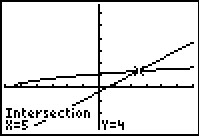### Finding Extraneous Solutions

In this activity, students will graphically solve a radical equation. They are given each step of solving the equation. For each step students are to graph each side of the equation as a separate function and find the intersection. Students will determine in which step the extraneous solution appears.
•TI-84 Plus CE
•TI-84 Plus C Silver Edition
• TI-84 Plus Silver Edition
• TI-84 Plus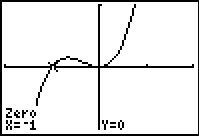### Going Back To Your Roots

Students apply the Fundamental Theorem of Algebra in determining the complex roots of polynomial functions.
•TI-84 Plus CE
•TI-84 Plus C Silver Edition
• TI-84 Plus Silver Edition
• TI-84 Plus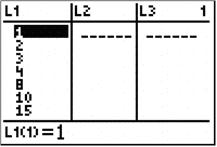### Investigation of End Behavior

Students use a scenario to begin their investigation of end behavior. In this scenario, students make a prediction about what they think will happen as more football players choose to order pizza. Students will hypothesize that the cost will go down as more players decide to order.
• TI-84 Plus Silver Edition
• TI-84 Plus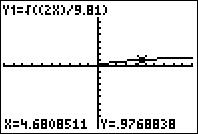### It's a Radical, Rational Universe!

Students explore values and optimization of rational and radical functions in real contexts by graphing and using spreadsheets.
•TI-84 Plus CE
•TI-84 Plus C Silver Edition
• TI-84 Plus Silver Edition
• TI-84 Plus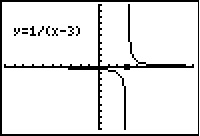### Rational Functions

Students investigate the graphs of functions of the form y = 1/(x - a). They will discover that the graph of such a function has a vertical asymptote at x = a, and a horizontal asymptote at y = 0. They will investigate the graphic and numeric consequences of such asymptotic behavior by observing a trace point on the graph move in response to various inputs for the independent variable.
•TI-84 Plus CE
•TI-84 Plus C Silver Edition
• TI-84 Plus Silver Edition
• TI-84 Plus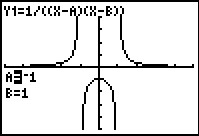### You Can't Get There From Here

Students will explore rational functions graphically and algebraically to identify singularities and asymptotes, both vertical and horizontal.
•TI-84 Plus CE
•TI-84 Plus C Silver Edition
• TI-84 Plus Silver Edition
• TI-84 Plus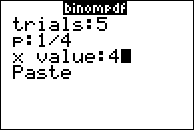••